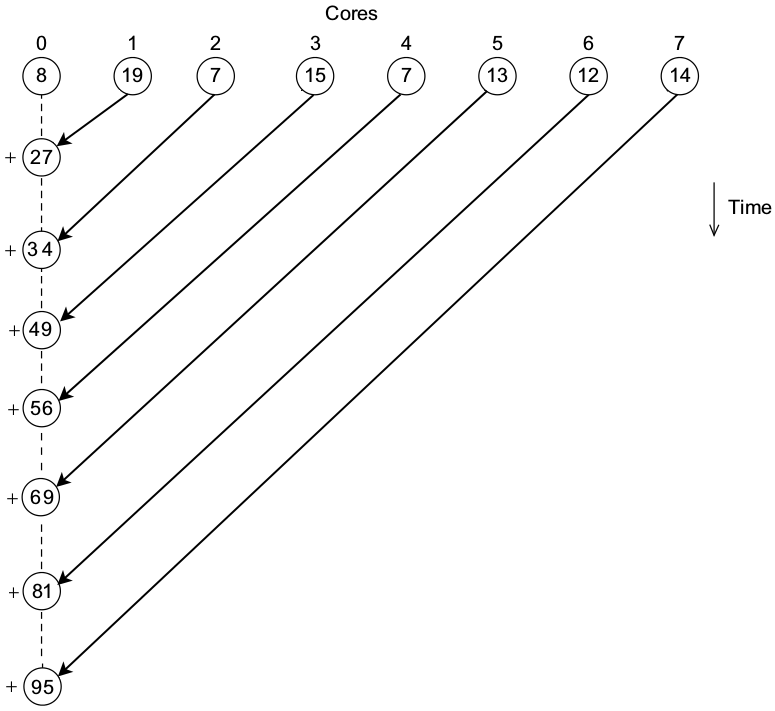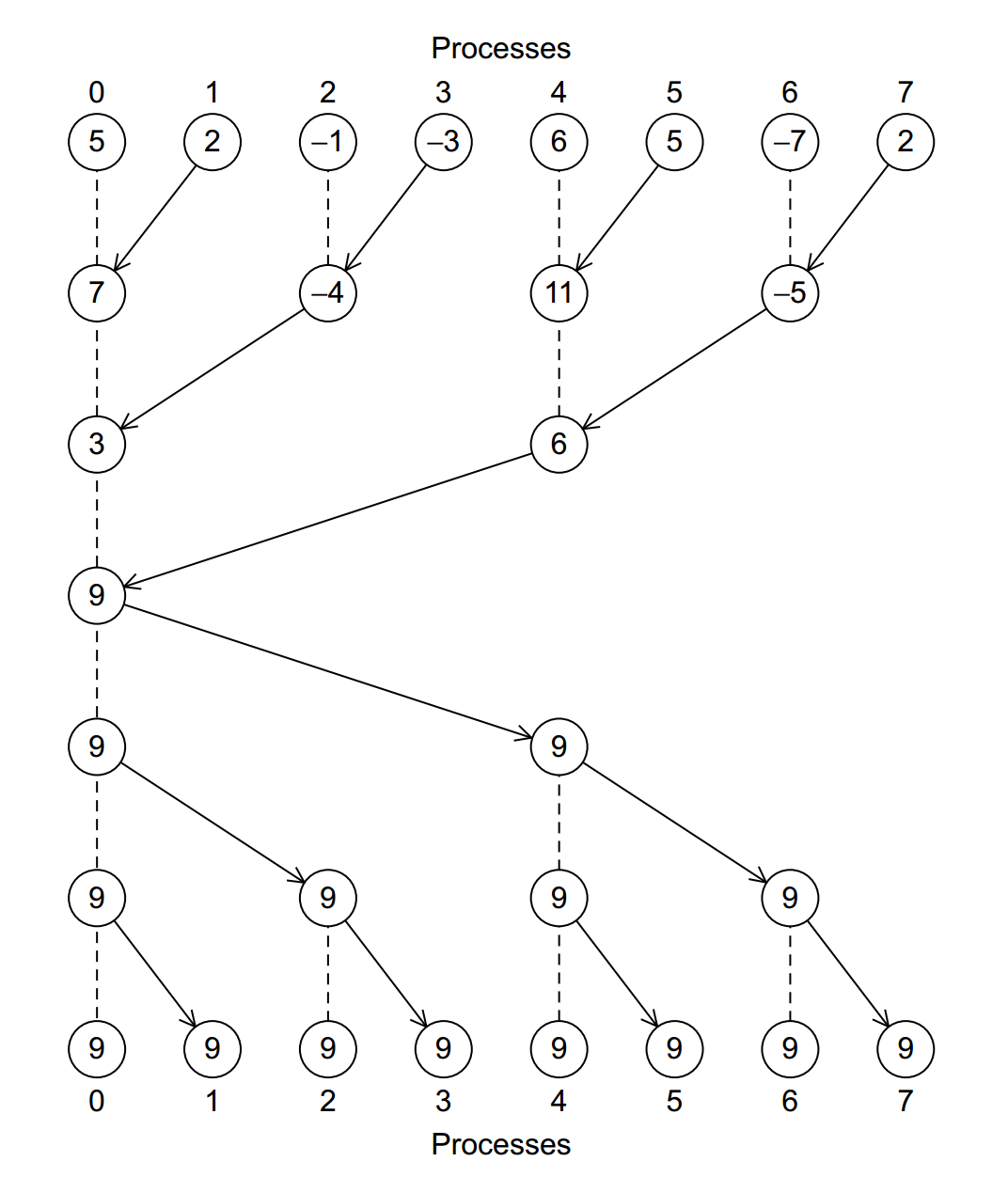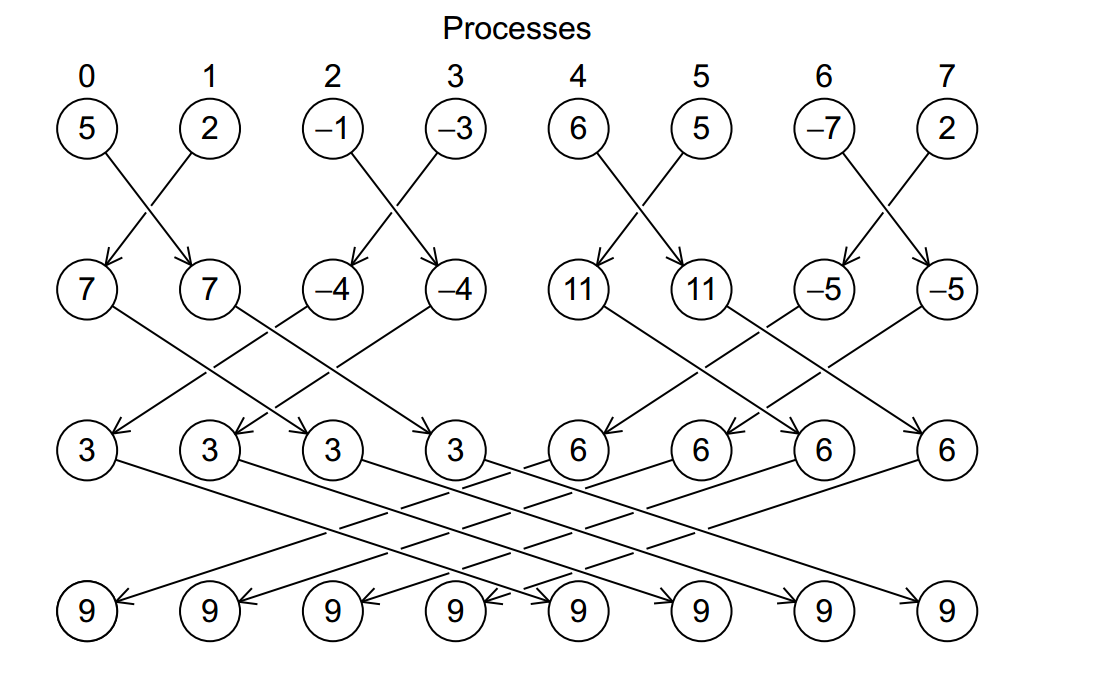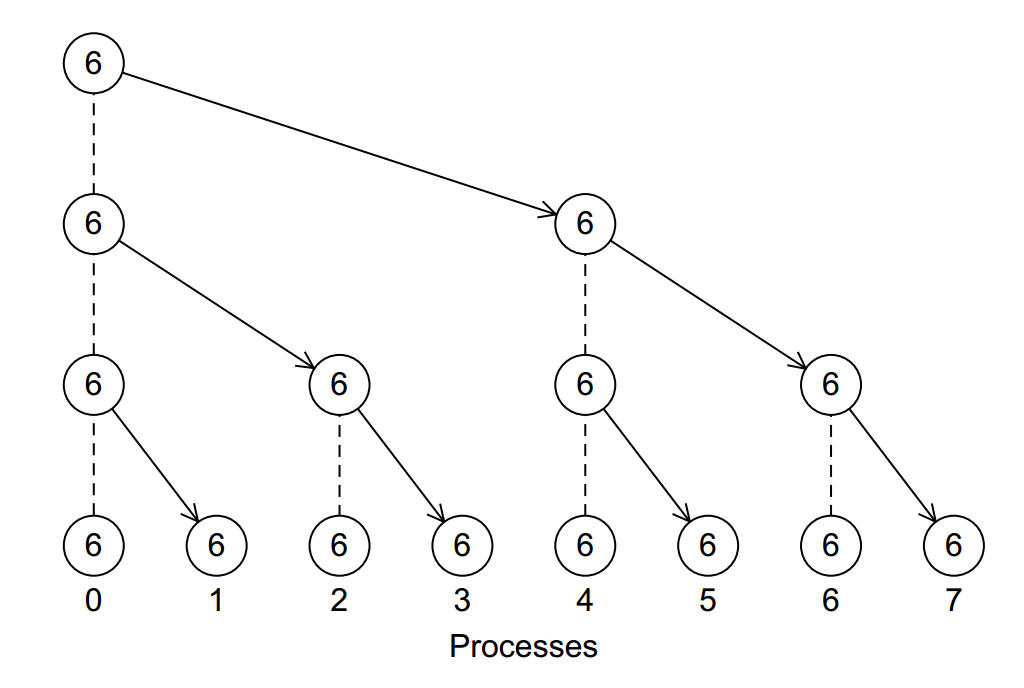# Collective Communication

## Overview

In the MPI sum program we looked at, all processes send their partial sum to process 0, who computes the final sum.

This means that process 0 must do $N - 1$ receive and addition operations:This can be done more intelligently by having the processes compute the global sum in a tree fashion:We could implement this with the point-to-point message passing we have seen, but this pattern is so common that MPI has support for this directly.

## MPI_Reduce

The global sum problem is an example of a reduction problem. We want to apply some operation across a set of data, and collect the result as one value.

MPI_Reduce is a collective communication function. It is used to specify that some communication should happen amongst all processes. The function looks like this:

The parameters are described below:

• sendbuf

A pointer to the piece of data this process is supplying. This is the partial sum in our example.

• recvbuf

A pointer to the memory location where the final answer should be stored.

• count

The number of elements in the send buffer.

• datatype

The type of element in the send buffer.

• op

The reduction operator to apply. This can be one of:

• MPI_MAX
• MPI_MIN
• MPI_SUM
• MPI_PROD
• MPI_LAND
• MPI_BAND
• MPI_LOR
• MPI_BOR
• MPI_LXOR
• MPI_BXOR
• MPI_MAXLOC
• MPI_MINLOC

• root

• comm

The communicator group.

## Sum Example

The program below uses MPI_Reduce to implement the parallel sum:


#include <stdlib.h>
#include <mpi.h>
#include <stdio.h>

#define START 0
#define END 100

int main(int argc, char** argv) {
int rank, size;

/* initialize MPI */
MPI_Init(&argc, &argv);

/* get the rank (process id) and size (number of processes) */
MPI_Comm_rank(MPI_COMM_WORLD, &rank);
MPI_Comm_size(MPI_COMM_WORLD, &size);

/* calculate the start and end points by evenly dividing the range */
int start = ((END - START) / size) * rank;
int end = start + ((END - START) / size) - 1;

/* the last process needs to do all remaining ones */
if (rank == (size - 1)) {
end = END;
}

/* do the calculation */
int sum = 0, i;
for (i = start; i <= end; i++) {
sum += i;
}

/* debugging output */
printf("Process %d: sum(%d, %d) = %d\n", rank, start, end, sum);

/* do the reduction */
int final;
MPI_Reduce(&sum, &final, 1, MPI_INT, MPI_SUM, 0, MPI_COMM_WORLD);

/* now have process 0 display the results */
if (rank == 0) {
printf("The final sum = %d.\n", final);
}

/* quit MPI */
MPI_Finalize();
return 0;
}


Note that this program is shorter, and should be more efficient as well.

## MPI_Reduce Caveats

• All the processes in the communicator group must call MPI_Reduce.
• The parameters must be compatible, i.e. the datatype, operator and root must match.
• The recvbuf is only used by the root process, but we still must pass an argument for each process.
• We can not use the same memory location for both the sendbuf and recvbuf.

## MPI_Allreduce

The MPI_Reduce function only gives one process the final result. This can be seen if we have each process print the sum:


/* do the reduction */
int final;
MPI_Reduce(&sum, &final, 1, MPI_INT, MPI_SUM, 0, MPI_COMM_WORLD);

printf("The final sum = %d.\n", final);


Sometimes, however, we may want all processes to have access to the final result. We could send the result to all processes using point to point communication, or we could use MPI_Allreduce instead:


int MPI_Allreduce(const void *sendbuf, void *recvbuf, int count,
MPI_Datatype datatype, MPI_Op op, MPI_Comm comm)


The function is identical to MPI_Reduce except that there is no root process - all processes receive the result.

MPI_Allreduce can be implemented with two tree structures:It can also have the processes exchange partial sums, which is sometimes called a butterfly:## MPI_Bcast

If we want to simply send a value from one process to all other processes, we can do that efficiently with a broadcast operation.This is done with the MPI_Bcast function:


int MPI_Bcast(void *data, int count, MPI_Datatype datatype, int root,
MPI_Comm comm)


The parameters are as follows:

• data

A pointer to a data buffer. For the process which is sending the data, this buffer is read from. For all other processes, it is written to.

• count

The number of items being broadcast.

• datatype

The type of data being broadcast.

• source

The rank of the process sending the data, all other processes receive.

• comm

The communicator group, which is often MPI_COMM_WORLD.

A common usage of MPI_Bcast is sending input values to all processes - since only process 0 can read from stdin. The following program demonstrates this.


#include <stdio.h>
#include <mpi.h>

int main(int argc, char** argv) {
int rank, size;

/* initialize MPI */
MPI_Init(&argc, &argv);

/* get the rank (process id) and size (number of processes) */
MPI_Comm_rank(MPI_COMM_WORLD, &rank);
MPI_Comm_size(MPI_COMM_WORLD, &size);

/* the data our program operatres on */
char string;

/* have process 0 read in a string */
if (rank == 0) {
printf("Enter a string: ");
fflush(stdout);
scanf("%s", string);
}

/* now broadcast this out to all processes */
MPI_Bcast(string, 100, MPI_CHAR, 0, MPI_COMM_WORLD);

/* print the string */
printf("Process %d has %s!\n", rank, string);

/* quit MPI */
MPI_Finalize();
return 0;
}


## Scatter & Gather

In many parallel programs, we want each process to work on a subset of an array. We could accomplish this by dividing the array into chunks, and sending each chunk to one of the processes. MPI provides a function just for this, however, MPI_Scatter:


MPI_Scatter(void* sendbuf, int sendcount, MPI_Datatype sendtype,
void* recvbuf, int recvcount, MPI_Datatype recvtype, int root, MPI_Comm comm);


The root process sends data while the others receive data.

The following program uses MPI_Scatter to split the input array amongst all processes:


#include <string.h>
#include <stdio.h>
#include <stdlib.h>
#include <mpi.h>

#define N 16

int main(int argc, char** argv) {
int rank, size;
int* data;

/* initialize MPI */
MPI_Init(&argc, &argv);

/* get the rank (process id) and size (number of processes) */
MPI_Comm_rank(MPI_COMM_WORLD, &rank);
MPI_Comm_size(MPI_COMM_WORLD, &size);

/* have process 0 read in the data */
if (rank == 0) {
printf("Enter %d values: ", N);
fflush(stdout);

data = malloc(N * sizeof(int));
for (int i = 0 ; i < N; i++) {
scanf("%d", data + i);
}
}

/* our portion of the data */
int portion[N / size];

/* now scatter the array to all processes */
MPI_Scatter(data, N / size, MPI_INT,
portion, N / size, MPI_INT, 0, MPI_COMM_WORLD);

/* print the data */
for (i = 0; i < (N / size); i++) {
printf("Process %d has %d!\n", rank, portion[i]);
}

/* quit MPI */
MPI_Finalize();
return 0;
}


Oftentimes, we will want to do the opposite: gather a portion of data from each process into one array in one process. This can be done with MPI_Gather:


int MPI_Gather(void *sendbuf, int sendcount, MPI_Datatype sendtype,
void *recvbuf, int recvcount, MPI_Datatype recvtype, int root, MPI_Comm comm)


This function takes exactly the same parameters as MPI_Scatter, but goes in the opposite direction. The following program extends the previous one to scatter data to all processes, have them square their data, then gather the results:


#include <string.h>
#include <stdio.h>
#include <stdlib.h>
#include <mpi.h>

#define N 16

int main(int argc, char** argv) {
int rank, size, i;
int* data;

/* initialize MPI */
MPI_Init(&argc, &argv);

/* get the rank (process id) and size (number of processes) */
MPI_Comm_rank(MPI_COMM_WORLD, &rank);
MPI_Comm_size(MPI_COMM_WORLD, &size);

/* have process 0 read in the data */
if (rank == 0) {
printf("Enter %d values: ", N);
fflush(stdout);

data = malloc(N * sizeof(int));
for (i = 0 ; i < N; i++) {
scanf("%d", data + i);
}
}

/* our portion of the data */
int portion[N / size];

/* now scatter the array to all processes*/
MPI_Scatter(data, N / size, MPI_INT,
portion, N / size, MPI_INT, 0, MPI_COMM_WORLD);

/* have each process square its data */
for (i = 0; i < (N / size); i++) {
portion[i] *= portion[i];
}

/* gather the data back up in process 0 */
MPI_Gather(portion, N / size, MPI_INT,
data, N / size, MPI_INT, 0, MPI_COMM_WORLD);

/* have process 0 print the results */
if (rank == 0) {
printf("Results: ");
for (i = 0; i < N; i++) {
printf("%d ", data[i]);
}
printf("\n");
}

/* quit MPI */
MPI_Finalize();
return 0;
}



There is also MPI_Allgather for situations where we want all processes to have the updated data array.